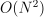# mlprodict#mlprodict was initially started to help implementing converters to ONNX. The main feature is a python runtime for ONNX. It gives more feedback than onnxruntime when the execution fails.

<<<

```import numpy
from sklearn.linear_model import LinearRegression
from mlprodict.onnxrt import OnnxInference
from mlprodict.onnxrt.validate.validate_difference import measure_relative_difference
from mlprodict import get_ir_version

X = iris.data[:, :2]
y = iris.target
lr = LinearRegression()
lr.fit(X, y)

# Predictions with scikit-learn.
expected = lr.predict(X[:5])
print(expected)

# Conversion into ONNX.
from mlprodict.onnx_conv import to_onnx
model_onnx = to_onnx(lr, X.astype(numpy.float32),
black_op={'LinearRegressor'},
target_opset=15)
print("ONNX:", str(model_onnx)[:200] + "\n...")

# Predictions with onnxruntime
model_onnx.ir_version = get_ir_version(15)
oinf = OnnxInference(model_onnx, runtime='onnxruntime1')
ypred = oinf.run({'X': X[:5].astype(numpy.float32)})
print("ONNX output:", ypred)

# Measuring the maximum difference.
print("max abs diff:", measure_relative_difference(
expected, ypred['variable']))

# And the python runtime
oinf = OnnxInference(model_onnx, runtime='python')
ypred = oinf.run({'X': X[:5].astype(numpy.float32)},
verbose=1, fLOG=print)
print("ONNX output:", ypred)
```

>>>

```    [0.172 0.343 0.069 0.059 0.034]
ONNX: ir_version: 8
producer_name: "skl2onnx"
producer_version: "1.13.1"
domain: "ai.onnx"
model_version: 0
doc_string: ""
graph {
node {
input: "X"
input: "coef"
output: "multiplied"
name
...
ONNX output: {'variable': array([[0.172],
[0.343],
[0.069],
[0.059],
[0.034]], dtype=float32)}
max abs diff: 6.303014714402957e-06
+ki='coef': (2, 1) (dtype=float32 min=-0.637811005115509 max=0.7347416877746582)
+ki='intercept': (1,) (dtype=float32 min=-1.3433398008346558 max=-1.3433398008346558)
+ki='shape_tensor': (2,) (dtype=int64 min=-1 max=1)
-- OnnxInference: run 3 nodes with 1 inputs
Onnx-MatMul(X, coef) -> multiplied    (name='MatMul')
+kr='multiplied': (5, 1) (dtype=float32 min=1.3775889873504639 max=1.6868011951446533)
+kr='resh': (5, 1) (dtype=float32 min=0.034249186515808105 max=0.34346139430999756)
Onnx-Reshape(resh, shape_tensor) -> variable    (name='Reshape')
+kr='variable': (5, 1) (dtype=float32 min=0.034249186515808105 max=0.34346139430999756)
ONNX output: {'variable': array([[0.172],
[0.343],
[0.069],
[0.059],
[0.034]], dtype=float32)}
```

These predictions are obtained with the following ONNX graph.

Notebook ONNX visualization shows how to visualize an ONNX pipeline. The package also contains a collection of tools to help converting code to ONNX. A short list of them:

• Python runtime for ONNX: `OnnxInference`, it is mostly used to check that an ONNX graph produces the expected output. If it fails, it fails within a python code and not inside C++ code. This class can also be used to call onnxruntime by using `runtime=='onnxruntime1'`. A last runtime `runtime=='python_compiled'` compiles a python function equivalent to code calling operator one by one. It makes easier to read the ONNX graph (see Execute ONNX graphs).

• Intermediate results: the python runtime may display all intermediate results, their shape if verbosity == 1, their value if verbosity > 10, see Execute ONNX graphs. This cannot be done with `runtime=='onnxruntime1'` but it is still possible to get the intermediate results (see `OnnxInference.run`). The class will build all subgraphs from the inputs to every intermediate results. If the graph has N operators, the cost of this will be.

• Extract a subpart of an ONNX graph: hen an ONNX graph does not load, it is possible to modify, to extract some subpart to check a tiny part of it. Function `select_model_inputs_outputs` may be used to change the inputs and/or the outputs.

• Change the opset: function `overwrite_opset` overwrites the opset, it is used to check for which opset (ONNX version) a graph is valid. …

• Visualization in a notebook: a magic command to display small ONNX graph in notebooks ONNX visualization.

• Text visualization for ONNX: a way to visualize ONNX graph only with text `onnx_text_plot`.

• Text visualization of TreeEnsemble: a way to visualize the graph described by a on operator TreeEnsembleRegressor or TreeEnsembleClassifier, see `onnx_text_plot`.

• Export ONNX graph to numpy: the numpy code produces the same results as the ONNX graph (see `export2numpy`)

• Export ONNX graph to ONNX API: this produces a a code based on ONNX API which replicates the ONNX graph (see `export2onnx`)

• Export ONNX graph to tf2onnx: still a function which creates an ONNX graph but based on tf2onnx API (see `export2tf2onnx`)

• Xop API: (ONNX operators API), see Xop API, most of the converting libraries uses onnx to create ONNX graphs. The API is quite verbose and that is why most of them implement a second API wrapping the first one. They are not necessarily meant to be used by users to create ONNX graphs as they are specialized for the training framework they are developped for.

• Numpy API for ONNX: many functions doing computation are written with numpy and converting them to ONNX may take quite some time for users not familiar with ONNX. This API implements many functions from numpy with ONNX and allows the user to combine them. It is as if numpy function where exectued by an ONNX runtime: Numpy to ONNX: Create ONNX graphs with an API similar to numpy.

• Benchmark scikit-learn models converted into ONNX: a simple function to benchmark ONNX against scikit-learn for a simple model: Measure ONNX runtime performances

• Accelerate scikit-learn prediction:, what if transform or predict is replaced by an implementation based on ONNX, or a numpy version of it, would it be faster? Speed up scikit-learn inference with ONNX

• Profiling onnxruntime: onnxruntime can memorize the time spent in each operator. The following notebook shows how to retreive the results and display them Profiling with onnxruntime.

This package supports ONNX opsets to the latest opset stored in mlprodict.__max_supported_opset__ which is:

<<<

```import mlprodict
print(mlprodict.__max_supported_opset__)
```

>>>

```    17
```

Any opset beyond that value is not supported and could fail. That’s for the main set of ONNX functions or domain. Converters for scikit-learn requires another domain, ‘ai.onnxml’ to implement tree. Latest supported options are defined here:

<<<

```import pprint
import mlprodict
pprint.pprint(mlprodict.__max_supported_opsets__)
```

>>>

```    {'': 17, 'ai.onnx.ml': 3}
```